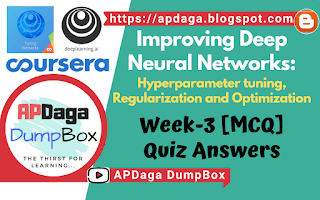# ▸Hyperparameter tuning, Batch Normalization, Programming Frameworks :Improving Deep Neural Networks Week-3 (MCQ)
Recommended Machine Learning Courses:

1. If searching among a large number of hyperparameters, you should try values in a grid rather than random values, so that you can carry out the search more systematically and not rely on chance. True or False?

• True

• False

1. Every hyperparameter, if set poorly, can have a huge negative impact on training, and so all hyperparameters are about equally important to tune well. True or False?

• True

• False
Yes. We’ve seen in lecture that some hyperparameters, such as the learning rate, are more critical than others.

1. During hyperparameter search, whether you try to babysit one model (“Panda” strategy) or train a lot of models in parallel (“Caviar”) is largely determined by:

• Whether you use batch or mini-batch optimization

• The presence of local minima (and saddle points) in your neural network

• The amount of computational power you can access

• The number of hyperparameters you have to tune

1. If you think β (hyperparameter for momentum) is between on 0.9 and 0.99, which of the following is the recommended way to sample a value for beta?

• r = np.random.rand()beta = r*0.09 + 0.9

• r = np.random.rand()beta = 1-10**(- r - 1)

• r = np.random.rand()beta = 1-10**(- r + 1)

• r = np.random.rand()beta = r*0.9 + 0.09

1. Finding good hyperparameter values is very time-consuming. So typically you should do it once at the start of the project, and try to find very good hyperparameters so that you don’t ever have to revisit tuning them again. True or false?

• True

• False

1. In batch normalization as presented in the videos, if you apply it on the lth layer of your neural network, what are you normalizing?

1. In the normalization formula , why do we use epsilon?

• To speed up convergence

• In case μ is too small

• To have a more accurate normalization

• To avoid division by zero

1. Which of the following statements about γ and β in Batch Norm are true?

• β and γ are hyperparameters of the algorithm, which we tune via random sampling.

• They set the mean and variance of the linear variable of a given layer.

• They can be learned using Adam, Gradient descent with momentum, or RMSprop, not just with gradient descent.

• The optimal values are , and β = μ.

• There is one global value of and one global value of for each layer, and applies to all the hidden units in that layer.

### Check-out our free tutorials on IOT (Internet of Things):

1. After training a neural network with Batch Norm, at test time, to evaluate the neural network on a new example you should:

• Skip the step where you normalize using μ and since a single test example cannot be normalized.

• If you implemented Batch Norm on mini-batches of (say) 256 examples, then to evaluate on one test example, duplicate that example 256 times so that you’re working with a mini-batch the same size as during training.

• Use the most recent mini-batch’s value of μ and to perform the needed normalizations.

• Perform the needed normalizations, use μ and estimated using an exponentially weighted average across mini-batches seen during training.

1. Which of these statements about deep learning programming frameworks are true?
(Check all that apply)

• A programming framework allows you to code up deep learning algorithms with typically fewer lines of code than a lower-level language such as Python.

• Deep learning programming frameworks require cloud-based machines to run.

• Even if a project is currently open source, good governance of the project helps ensure that the it remains open even in the long term, rather than become closed or modified to benefit only one company.

&
Click here to see more codes for Raspberry Pi 3 and similar Family.
&
Click here to see more codes for NodeMCU ESP8266 and similar Family.
&
Click here to see more codes for Arduino Mega (ATMega 2560) and similar Family.

Feel free to ask doubts in the comment section. I will try my best to answer it.
If you find this helpful by any mean like, comment and share the post.
This is the simplest way to encourage me to keep doing such work.

Thanks & Regards,
- APDaga DumpBox